Question

A horizontal piston/cylinder arrangement is placed in a constant temperature bath. The piston slides in the cylinder with negligible friction, and an external force hold it in place against an initial gas pressure of 1 bar. The initial gas volume is 0.04 m^3. The external force on the piston is reduced gradually, and the gas expands isothermally as its volume triples.

(a) If the volume of the gas is related to its pressure so that the product PV^t is constant, what is the work done by the gas in moving the external force?

(b) How much would be done if the external force were suddenly reduced to half its initial value instead of being gradually reduced?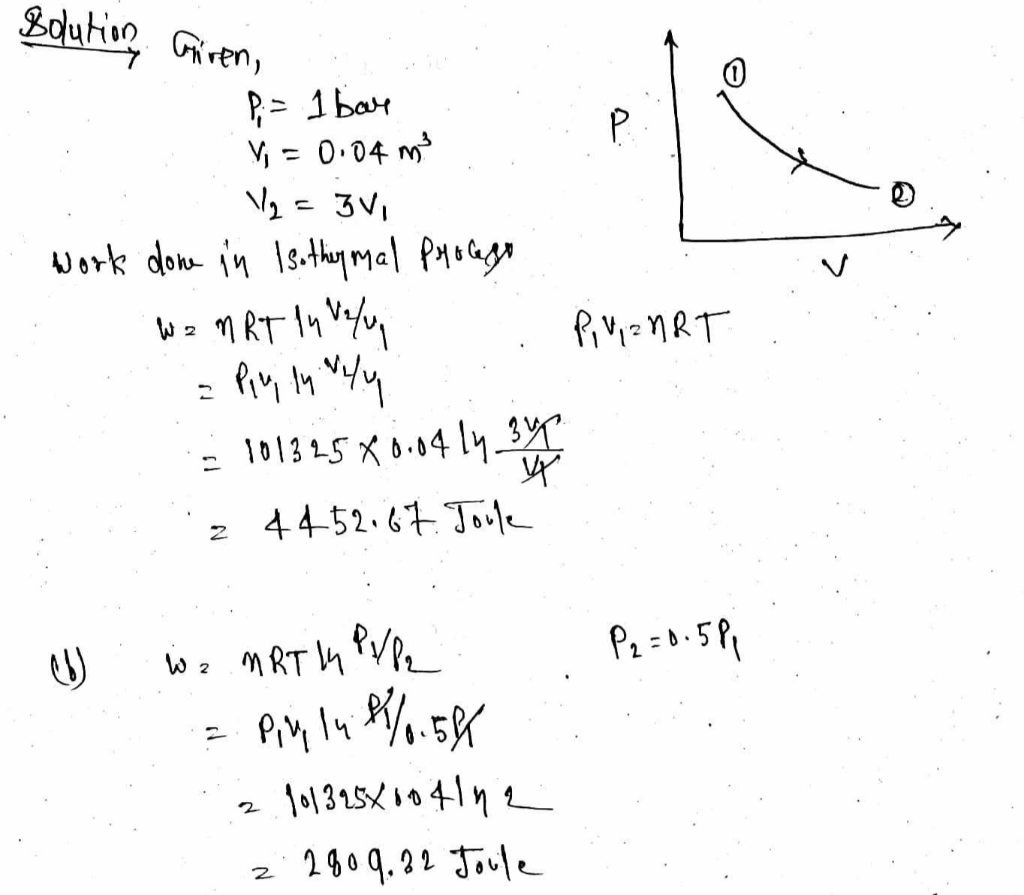#### Earn Coins

Coins can be redeemed for fabulous gifts.

Similar Homework Help Questions
• ### A horizontal piston/cylinder arrangement is placed in a constant-T bath. The piston slides in the cylinder...A horizontal piston/cylinder arrangement is placed in a constant-T bath. The piston slides in the cylinder with negligible friction, and an external force holds it in place against an initial gas pressure of 16 bar. The initial gas volume is 0.04 m^3. The external force on the piston is reduced gradually, and the gas expands isothermally as its volume doubles. If the volume of the gas is related to its pressure so that the product PV^t is constant, what is...

• ### 5. Consider the following piston-cylinder arrangement which is thermally insulated. Initially, the same 1 mole of...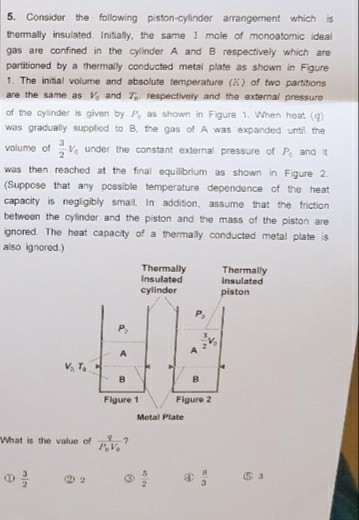5. Consider the following piston-cylinder arrangement which is thermally insulated. Initially, the same 1 mole of monoatomic ideal gas are confined in the cylinder A and B respectively which are partitioned by a thermally conducted metal plate as shown in Figure 1. The initial volume and absolute temperature (X) of two partitions are the same as V and T respectively and the external pressure of the cylinder is given by P, as shown in Figure 1. When heat (g) was...

• ### A cylinder with a moveable piston holds 2.90 Mol of argon at a constant temperature of 255K . As...

A cylinder with a moveable piston holds 2.90 Mol of argon at a constant temperature of 255K . As the gas is compressed isothermally, its pressure increases from 105kPato 123kPa .Find the final volume of the gas.Find the work done by the gas.Find the heat added to the gas.

• ### Carbon dioxide contained in a piston-cylinder arrangement, initially at 6 bar and 400K, undergoes an expansion...

Carbon dioxide contained in a piston-cylinder arrangement, initially at 6 bar and 400K, undergoes an expansion to a final temperature of 298 k, during which the pressure-volume relationship if pV^1.2 = constant. Assuming the ideal gas model for the CO2, determine the final pressure, in bar, and the work and heat transfer, each in kJ/kg

• ### P3A.2 A sample consisting of 0.10 mol of perfect gas muICLUL is piston inside a cylinder...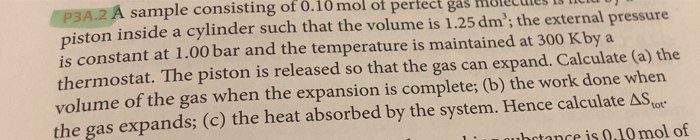P3A.2 A sample consisting of 0.10 mol of perfect gas muICLUL is piston inside a cylinder such that the volume is 1.25 dm'; the external pressure is constant at 1.00 bar and the temperature is maintained at 300 K by a thermostat. The piston is released so that the gas can expand. Calculate (a) the volume of the gas when the expansion is complete; (b) the work done when the gas expands; (c) the heat absorbed by the system. Hence...

• ### 4) The work produced by a piston-cylinder device depends on the pressure inside the cylinder and ...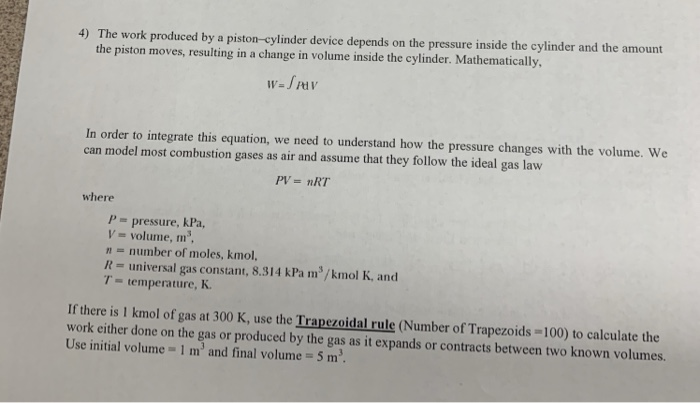visual studio 2017 c++ 4) The work produced by a piston-cylinder device depends on the pressure inside the cylinder and the amount the piston moves, resulting in a change in volume inside the cylinder. Mathematically In order to integrate this equation, we need to understand how the pressure changes with the volume. We can model most combustion gases as air and assume that they follow the ideal gas law PV- nRT where P- pressure, kPa, volume, m n- number of...

• ### A 19.0- L volume of an ideal gas in a cylinder with a piston is at...

A 19.0- L volume of an ideal gas in a cylinder with a piston is at a pressure of 3.2 atm . Enough weight is suddenly removed from the piston to lower the external pressure to 1.6 atm . The gas then expands at constant temperature until its pressure is 1.6 atm . The change in the internal energy of a system, ΔE , for an isothermal expansion of an ideal gas is 0. Use this information in your calculations...

• ### Air is trapped in a piston cylinder arrangement. The air expands from a temperature of 60...

Air is trapped in a piston cylinder arrangement. The air expands from a temperature of 60 C and a pressure of 280 KPa to a pressure of 140 KPa. During the process, 30 KJ/Kg of work is done and 14 KJ/Kg of heat is removed. The initial volume is 0.00878 m^3. a) What is the mass of the air? b) What is the temperature change during this process? c) What is the entropy change during this process? d) Does the...

• ### Air contained within a vertical piston-cylinder assembly is shown in Figure 1. On its top, the 10-kg piston is attached to a spring and exposed to an atmospheric pressure of 1 bar. Initially, the bottom of the piston is at x = 0, and the spring exerts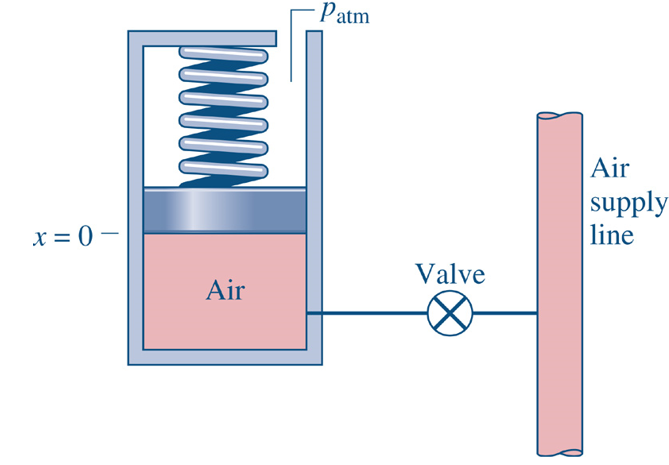Air contained within a vertical piston-cylinder assembly is shown in Figure 1.         On its top, the 10-kg piston is attached to a spring and exposed to an     atmospheric pressure of 1 bar. Initially, the bottom of the piston is at x = 0, and         the spring exerts a negligible force on the piston. The valve is opened and air     enters the cylinder from the supply line, causing the volume of the air within the             cylinder...

• ### 2 kg Water in a vertical piston cylinder arrangement, is initially at saturated liquid state at...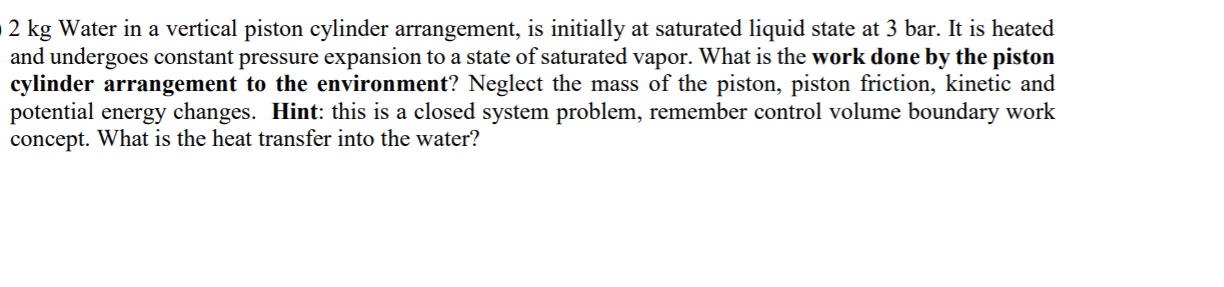2 kg Water in a vertical piston cylinder arrangement, is initially at saturated liquid state at 3 bar. It is heated and undergoes constant pressure expansion to a state of saturated vapor. What is the work done by the piston cylinder arrangement to the environment? Neglect the mass of the piston, piston friction, kinetic and potential energy changes. Hint: this is a closed system problem, remember control volume boundary work concept. What is the heat transfer into the water?